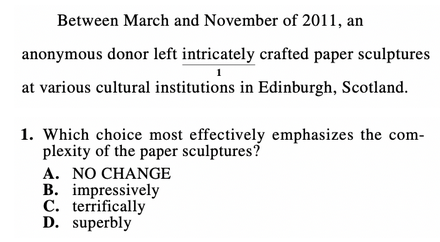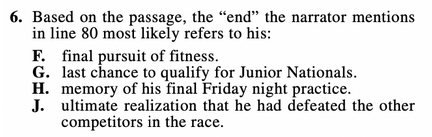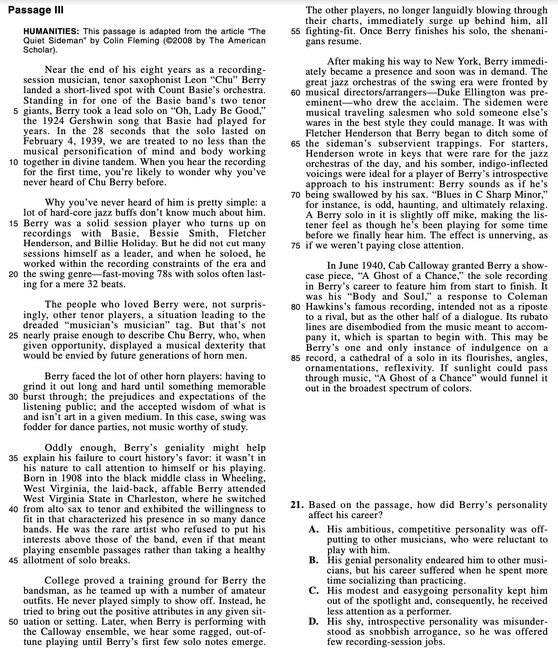### What are your chances of acceptance?

Duke University
UCLA
Unweighted GPA: 3.7
1.0
4.0
SAT: 720 math
200
800
| 800 verbal
200
800

#### Extracurriculars

Low accuracy (4 of 18 factors)Nisha Desai
7

# How to Prepare for the PreACT + Practice Questions

### Do you know how to improve your profile for college applications?

See how your profile ranks among thousands of other students using CollegeVine. Calculate your chances at your dream schools and learn what areas you need to improve right now — it only takes 3 minutes and it's 100% free.

## What’s Covered:

The PreACT is a standardized test that students usually take before the ACT. This test is multiple-choice and contains a format similar to the ACT, but with less questions and no writing section. It’s usually a good indicator of how you’ll do on the ACT (your PreACT score report even includes a predicted score for the ACT), so you should make sure to try your best when taking it.

## How to Prepare for the PreACT

### Utilize practice tests

While there are no official PreACT practice tests, the questions on the PreACT are of similar format (but slightly easier) as those on the ACT. So, you can prepare for the PreACT by taking official ACT practice tests. As you take these tests, keep in mind that the PreACT is shorter (in terms of the number of questions and the time allotted for each section) and has no writing test.

So, try to time yourself with the format of the PreACT in mind as you take practice tests. Also, make sure to take the time to grade your test, paying special attention to where you’re making the most mistakes so that you can become more cognizant of your weaknesses in order to improve them.

As mentioned earlier, taking practice tests is a great way to pinpoint where your weaknesses lie. Try to notice patterns of incorrect responses so that you know which issues need to be addressed. For example, if you find yourself consistently missing geometry questions on the math section, take the time to learn geometry and focus on those types of questions.

As you read, try asking yourself reading comprehension questions, or summarizing what you just read. Also, practice active reading. This involves underlining, circling, or even jotting down notes in the margin. These practices will make answering questions on the reading section much easier, since you’ll already know the important information and main ideas.

### Practice time management

Time management skills are essential for any standardized test. Make sure to time yourself as you take practice tests to simulate the testing environment. This way, you’ll be prepared when it’s time to take the actual PreACT.

If you find yourself running out of time, try skipping harder questions to see if that helps you score better. Alternatively, if you find yourself having extra time, yet making careless mistakes, learn to go back and check your work, or take more time on questions that are fairly calculation-heavy or require careful reading.

### Guess

When all else fails, guess! If you’re down to the last few minutes during the test and still have some unanswered questions, it’s important to guess rather than leave any questions blank. This is because the PreACT, similar to the ACT, has no penalty for incorrect answers. It’s in your best interest to guess as a last resort.

Also, if you find yourself taking too long on a particular question, make an educated guess, then come back to it if you have the time. Since questions carry equal weight, spending the same amount of time on three easy questions is more beneficial than on one harder question.

## Discover your chances at hundreds of schools

Our free chancing engine takes into account your history, background, test scores, and extracurricular activities to show you your real chances of admission—and how to improve them.

## PreACT Practice Questions

All of the following questions are taken from official ACT practice tests and are likely representative of what you’ll see on the actual PreACT.

### Question 1 (English)The ACT English test expects you to know grammar conventions. This question tests common misconceptions with subject-verb agreement.

We can start with subject-verb agreement to eliminate answer choices. Choices A and D are incorrect since “horse” is a singular noun, but “leap” is a plural verb, which makes “horses leap” grammatically incorrect. We can correct this error in two ways:

1. “As horses leap”
2. “As a horse leaps”

This means that only answer choice C is correct. Answer choice B is incorrect because the phrase “horse’s leaps” indicates a possessive. This means that the leaps belong to the horse, which is not what the sentence is trying to communicate.

### Question 2 (English)Along with grammar, you’ll also be tested on writing style and word choice. For this question, we’ll need to determine which adverb best communicates the complexity of the sculptures. Answer choices B, C, and D all emphasize that the sculptures are great, but don’t really communicate that they’re complex.

So, answer choice A is the correct option in this scenario. These types of questions involve understanding the connotations of different words. Unlike questions that test your grammar skills, there’s no formulaic way to answer questions involving word choice. So, a great way to develop your skill set is by reading; this way, you’ll familiarize yourself with how vocabulary is used in context, making these questions much easier to answer.

### Question 3 (Math)Let’s start by defining some variables: let $$h$$ be the amount of home games Armin attends. Then, the total cost of buying an individual ticket for each game is $$14h$$, since each ticket costs $14. So, if we want the season pass to cost less than the total cost of buying individual tickets, we have the following inequality with which we can solve for $$h$$: $$175\lt 14h$$ $$12.5\lt h$$ We’ll need to round up in this case, because if Armin attends 12 games, the total cost is $$14(12)=$$168$, which is less than $175. So, we’ll need to round up to 13 games. If you want to stay away from algebra you can also test out the answer choices, starting with the lowest choice, since you are looking for the minimum number of games. $$14\cdot 13=182$$. This is greater than$175, so the season pass would be cheaper if Armin attends 13 games.

### Question 4 (Math)Again, we can start by defining variables. Let $$x$$ represent the lesser number and let $$y$$ represent the greater number. Now, we can look at the given information.

If the sum of the 2 numbers is 151, we have that: $$x+y=151$$.

Next, the second sentence gives us the following equation: $$x=19+\sqrt{y}$$. So, we can substitute the second equation into the first:

$$(19+\sqrt{y})+y=151$$

$$\sqrt{y}+y=132$$

$$y+\sqrt{y}-132=0$$.

We can solve this by factoring, but to make it easier, we can substitute. Let $$a=\sqrt{y}$$. Then, our equation becomes as follows:

$$a^2 + a – 132 = 0$$

If we factor this, we get that $$(a-11)(a+12)=0$$.

Then, we have that $$a=11$$ or $$a=-12$$. Since $$a=\sqrt{y}$$, we must have that $$a$$ is positive, so $$a=11$$. Then, $$y=121$$. We can use the first equation to solve for $$x$$:

$$x+121=151$$

$$x=30$$

But, we’re not done here. We need to find the difference between $$y$$ and $$x$$:

$$y-x=121-30=91$$.Though this question refers to line 80, we’ll actually need to look to the beginning of the passage for the right answer. The passage opens with the speaker indicating that they were trying to qualify for the Junior Nationals. Later on, in lines 8–11, the speaker mentions that they had one more final chance to qualify, indicating that G is the correct answer.

But, to make sure, let’s check the other answer choices. Options F and H are incorrect since the speaker doesn’t mention that they will no longer have Friday night practices or that they would no longer swim. Also, option J is incorrect since the speaker mentions in line 23 that they’re the only one in the race, so there are no other competitors.For this question, we’ll need to look at the fifth paragraph, which states that “Berry’s geniality might help explain his failure to court history’s favor: it wasn’t in his nature to call attention to himself or his playing.”

If you fail to read carefully, this might direct you towards option B, which correctly refers to Berry’s genial personality. However, the explanation for option B is incorrect—it claims that Berry’s failure was due to prioritizing socializing over practicing. But, the passage states that Berry simply didn’t want to call attention to himself, which emphasizes his modesty. This means that option C is the correct answer.

### Question 7 (Science)For this question, we can actually look to the graphs for the correct answer. We see that in the graph for Experiment 1, the axes are labelled with volume and mass. This means that neither volume nor mass were kept constant during experiment one, and thus answer choices B and D are incorrect.

Next, we can look at the graph for Experiment 2. The axes are labelled with volume and temperature, so answer choice C is also incorrect.

Then, we are left with atmospheric pressure. This is consistent with the information in the passage, which says “The atmospheric pressure was 758 mm Hg throughout all 3 steps.”

### Question 8 (Science)This question requires us to ignore prior knowledge. So, instead of using our own reasoning, we’ll need to look at Student 1’s reasoning. Student 1 claims that two samples need to have the same values for all five properties to be the same substance.

This means that we’ll need to look for which two samples have equal values for all five properties. If we look at samples C and D, we can see that they have the same mass, volume, density, melting point, and boiling point, so answer choice C is the correct answer.

## How Does the PreACT Affect My College Chances?

The PreACT actually doesn’t have a direct impact on your admissions chances. You won’t be asked to submit your PreACT score on your college applications. But, the PreACT is helpful because it is a good predictor of your success on the actual ACT. Your PreACT score breakdown can help you realize what areas to focus on and give you an estimate of how you’ll do on test day.

Do you want to see how your actual or predicted ACT scores affect your college chances? Try out CollegeVine’s free Admissions Calculator. This tool utilizes your test scores, GPA, extracurriculars and more to determine your chances at the schools that you’re interested in. Then CollegeVine offers tips on how to improve your profile so that you can become an even more competitive applicant!Short Bio
Nisha Desai is a second year student at the University of California, Riverside. She recently started working at CollegeVine, but has done application guidance and tutoring in a private capacity for a couple of years. She is in school to eventually get her Masters in Education and enjoys reading and running in her free time.